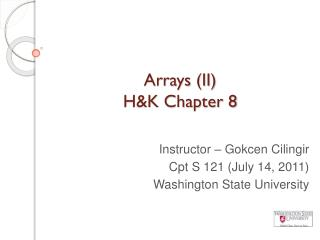DownloadDownload PresentationArrays (II) H&K Chapter 8

# Arrays (II) H&K Chapter 8

Télécharger la présentation## Arrays (II) H&K Chapter 8

- - - - - - - - - - - - - - - - - - - - - - - - - - - E N D - - - - - - - - - - - - - - - - - - - - - - - - - - -
##### Presentation Transcript

1. Arrays (II) H&K Chapter 8 Instructor – GokcenCilingir Cpt S 121 (July 14, 2011) Washington State University

2. Array Searching • Lots of motivating problems • Find a name in the phone book • Find a student in the class list • Others?

3. Sequential Search Algorithm • Solution strategy: search list sequentially until we find the target or until we’re sure that target is not in the list Get the value of target and the list of values Set the value of index to 0 Set the value of found to false While (index < n) and (found == false) if target = list[index] set found to true else set index to index + 1 Endwhile Note how we stop once we find the target.

4. Sequential search implementation with C Indicates it’s a strictly input parameter intsequential_search( constintvalues[], inttarget, intn) { intindex, found; index = 0; found = 0; // false //search until found or end of array while (index < n && !found) { if (values[index] == target) // We've found the target found = 1; else // Keep looking index++; } //Handle the result of the search if (found) // return index at which target was found return index; else // return NOT_FOUND flag return NOT_FOUND; // #defined as -1 }

5. Sequential search on a sorted array • If the input to sequential search algorithm was a sorted array (ascending order), we would change our loop as follows to save from execution time: while (index < n ) { if (values[index] == target) return index; // We've found the target else if (values[index] > target) return NOT_FOUND; //We’re sure target is not there else // Keep looking index++; } return NOT_FOUND; • We can save much more from execution time by using binary search algorithm.

6. Binary Search Algorithm • Input: a list of n sorted values and a target value • Output: True if target value exists in the list and location of target value, false otherwise • Method: • Set left to 0 and right to n-1 • Set found to false • While found is false and left is less than or equal to right • Set mid to midpoint between left and right • If target == item at mid then set found to true • If target < item at mid then set right to mid – 1 • If target > item at mid then set to left to mid + 1 • If found == true then print “Target found at location mid” • Else print “Sorry, target value could not be found.”

7. Binary Search Algorithm(2) • Idea is to narrow down the search space by half until either we find the target or we cannot narrow down the space any more which means target is not in the list • In the beginning, our search space is the whole array • During the search, we’re going to keep track of the narrowed search space (which will be a sub-array) by advancing the beginning and the end index of our “logical” search space.

8. Binary Search Visualization • Check out this applet to visualize the discussed binary search algorithm.

9. Binary search implementation with C intbinary_search( const intvalues[], int target, intnum_values) { intfound, left, right, mid; found = 0; // false left = 0; right = num_values; while (!found && left <= right) { mid = (left + right)/2; if (target == values[mid]) found = 1; // true else if (target < values[mid]) // target < values[mid] right = mid - 1; else // target > values[mid] left = mid + 1; } if (found) return mid; else return NOT_FOUND; // #defined as -1 }

10. Array Sorting (1) • Lots of motivating problems • Print out a class list in alphabetical order • Order finish times in a race to determine who placed • Make look-up easier (preparation for binary search)

11. Selection Sort Algorithm • Input: a list of numbers • Output: a list of the same numbers in ascending (increasing) order • Method: • Set marker that divides “sorted” and “unsorted” sections of list to the beginning of the list • While the unsorted section of the list is not empty • Call upon Find_Smallest helper function to find index of the smallest value in “unsorted” section of list • Swap smallest value in "unsorted section of list" with first value in “unsorted” section of list • Move "sorted/unsorted" marker to right one position

12. Selection Sort Visualization • Check out this applet to visualize the discussed selection sort algorithm.

13. Selection sort implementation with C void selection_sort(int values[], intnum_values) { intmarker, index_of_smallest, temp; for (marker = 0; marker < num_values - 1; marker++) { /* Find the index of the smallest element in unsorted list*/ index_of_smallest = find_smallest(values,marker,num_values-1); /* Swap the smallest value in the subarray i+1 .. num_values - 1 with the value[i], thereby putting into place of the ith element. */ temp = values[marker]; values[marker] = values[index_of_smallest]; values[index_of_smallest] = temp; } } intfind_smallest(int values[], int low, int high) { intsmallest_index, i; smallest_index = low; for (i = low + 1; i <= high; i++) if (values[i] < values[smallest_index]) smallest_index = i; return smallest_index; }

14. References • J.R. Hanly & E.B. Koffman, Problem Solving and Program Design in C (6th Ed.), Addison-Wesley, 2010 • P.J. Deitel & H.M. Deitel, C How to Program (5th Ed.), Pearson Education , Inc., 2007.